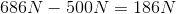# AP Physics B : Understanding Normal Force

## Example Questions

### Example Question #1 : Understanding Normal Force

A 2kg box is at the top of a frictionless ramp at an angle of 60o. The top of the ramp is 30m above the ground. The box is sitting still while at the top of the ramp, and is then released.

If the angle of the ramp is decreased, which of the following statements is false?

The time to reach the ground will increase

The force due to gravity will decrease

The net force on the box will decrease

The normal force will decrease

The normal force will decrease

Explanation:

When given a question about the angle of a ramp, compare it to the extreme angles: 0o and 90o.

1. When the ramp has an angle of 0o, the net force 0. The force due to gravity must equal the normal force; thus the normal force is at a maximum value.

2. When the angle of the ramp is 90o, the full force of gravity is experienced by the box, and there is no normal force. The net force is equal to the force of gravity. Remember that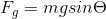, so then theta is 90o, force of gravity is at a maximum.

When we decrease the angle of the ramp, we get closer to scenario 1. As a result, we can conclude that the normal force on the box increases, rather than decreases.

### Example Question #2 : Understanding Normal Force

A woman is standing on a scale in an elevator as it accelerates upward. The elevator then stops accelerating and continues upward at a constant speed. Which of the following statements is true?

According to the scale, the woman's weight did not change throughout the motion

According to the scale, the woman weighed more when the elevator was moving at a constant speed

According to the scale, the woman weighed less when the elevator was accelerating

According to the scale, the woman weighed more when the elevator was accelerating

We must know the woman's mass to draw any conclusions

According to the scale, the woman weighed more when the elevator was accelerating

Explanation:

The normal force of the woman is measured by the scale. While accelerating upward, the scale should read a larger weight than when it is at rest. When the elevator is moving upward at a constant speed, the scale should read the same as when it is at rest. This is because the normal force is generated to counter the downward forces pushing against the floor. When the elevator is accelerating, there is a net upward force from the acceleration as well as the normal force to counter gravity. The normal force generates an upward acceleration. When moving at a constant speed, there is no upward acceleration and the normal force acts only to counter gravity. The normal force, and scale reading, will thus be greater during the period of acceleration.

### Example Question #3 : Understanding Normal Force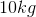block is placed on aincline. What is the normal force of the incline on the box?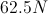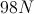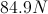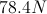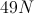Explanation:

To find the normal force on the incline, we use the relationship: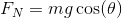This provides the magnitude of the force of gravity in the direction perpendicular to the incline. Normal force will always act in the direction perpendicular to the surface, and in this case will be equal and opposite to the force of gravity. We then plug in the mass and gravitational acceleration to find the normal force on this block: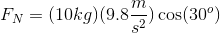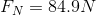### Example Question #4 : Understanding Normal Force

Five substitute players on a basketball team are sitting on the bench during a game. The bench weighs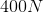and, altogether, the players weigh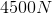. Two players, weighing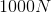and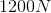, stand up. What is the difference in the normal force acting on the bench before and after these players leave?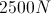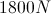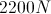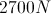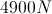Explanation:

The normal force is generated as a result of a force against a solid surface. As per Newton's third law, the surface will exert an equal and opposite force on the object in contact. If an object is resting on a flat surface, then the normal force will be working to counter the weight of the object due to gravity.

The normal force acting on the bench with five players is equal and opposite to the total weight of the bench and players. Keep in mind that weight acts in the downward direction.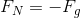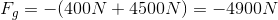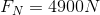When the two players stand up, the new normal force is reduced.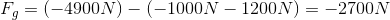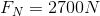The difference in the normal force is: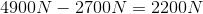We could also have found this change by adding the weights of the two players who stood.

### Example Question #5 : Understanding Normal Force

A person tries to lift a very heavy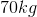rock by applying an upward force of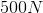, but is unable to move it upward. Calculate how much additional force was needed to lift the rock from the ground.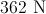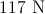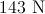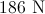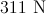Explanation:

First, calculate the gravitational force acting on the rock.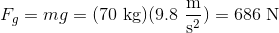The exerts a force of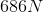downward, meaning that if the person exerted at least, then he or she would have been able to lift it up. Instead, the person applied only. This means that the person needed to apply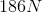of additional force to lift the rock.